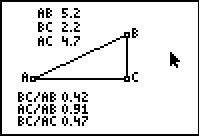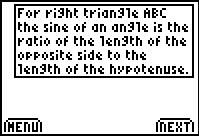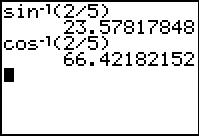••• ##### Device
• TI-83 Plus Family
• TI-84 Plus
• TI-84 Plus Silver Edition

TI Connect™

• ##### Report an Issue

Geometry: Ratios of Right Triangles
by Texas Instruments#### Overview

In this activity, students will explore the ratios of right triangles. Students will discover that we can find the measure of the angles of a right triangle given the length of any two sides.

#### Key Steps

•In this activity, students will investigate ratios of right triangles. First, students will be asked several questions about the definition of the sine, cosine, and tangent ratios.

•Next, students will investigate the sine and cosine ratios. Since the ratios are fixed in a right triangle, they can find the measures of the angles using trigonometry. Students will use the Cabri Jr. application to collect data and see that the ratio remains constant.

•Finally, the students will be asked to apply what they have learned to find the measure of the angles of a right triangle given the measure of at least two sides.